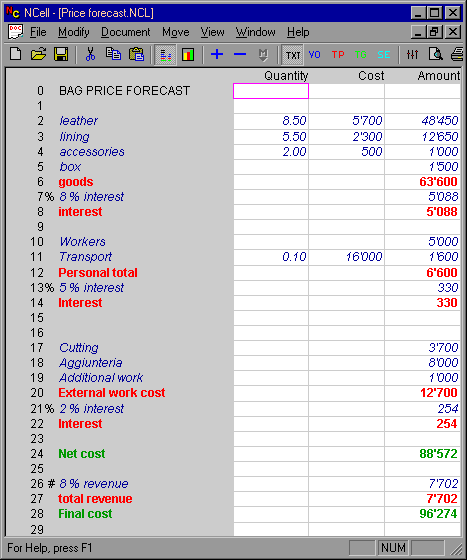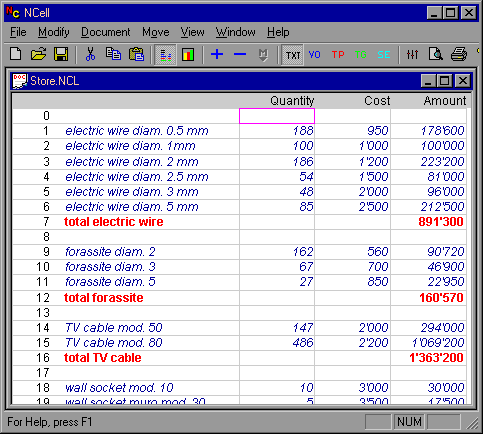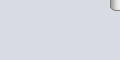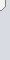# Example of price forecast with NCellIn this example you can see some interesting features of NCell:

Multiplying columns

Columns "Quantity" and "Cost" are set as multiplyng, while "Amount" is standard, so that when "Quantity x Cost" is different from 0 the result is placed in "Amount", while when the product is 0 the value to add can be inserted in "Amount" directly.

% Rows

Rows 7, 13 and 21 are %rows so they contain the % value of the previous non text line, in case of row 7 the value is 7% of goods.

delta % Rows
Like the previous example row 26 contain 8% of line 28.

## Example of inventory for a store with NCellThis is a simple example where you can see multiplying colums "Quantity" and "Cost", the result is placed in amount, which also contain the result of the sum of the various groups.EnglishItaliano

AL-TEK 2016 twin site Development and consulting